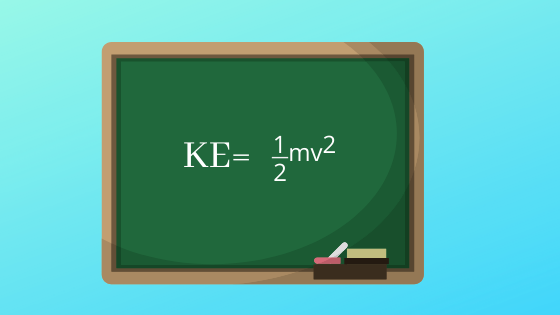# Kinetic Energy CalculatorNote
• Enter the values of the two known variables in the text boxes
• Leave the text box empty for the variable you want to solve for
• Click on the calculate button.

Formula used
$K=\frac {1}{2} mv^2$

## What is Kinetic Energy

Kinetic energy is the energy possessed by the body by virtue of its motion. Kinetic Energy of the body as one half of the product of mass of the body and the square of its Speed It is a Scalar quantity and SI unit is Joule. Kinetic Energy is given by the formula
$K = \frac {1}{2} mv^2$
Where
K-> Kinetic Energy
m -> Mass of the object
v-> Velocity of the object

Example of Few questions where you can use this formula
Question 1
Calculate the Kinetic energy of the object have mass 10 kg and velocity 3m/s
Solution
m=10 kg, v= 3m/s , K=?
Kinetic Energy is given by the formula
$K = \frac {1}{2} mv^2$
$K= \frac {1}{2} \times 10 \times 3^2 = 45 \ J$

Question 2
An Kinetic energy is 10 J.If the object mass is 1 kg ,calculate the velocity of the object?
Solution
m=1 kg, v= ? , K=10J
Kinetic Energy is given by the formula
$K = \frac {1}{2} mv^2$
Rearranging
$v= \sqrt { \frac {2K}{m}} = \sqrt {\frac {2 \times 10}{1}} = \sqrt {20} = 2 \sqrt 5 \ m/s$

Question 3
An Kinetic energy is 36 J.If the velocity is 3 m/s ,calculate the mass of the object?
Solution
m=?, v= 3 m/s , K=36J
Kinetic Energy is given by the formula
$K = \frac {1}{2} mv^2$
Rearranging
$m = \frac {2K}{v^2} = \frac {2 \times 36}{3^2} = 8 \ kg$

## How the Kinetic Energy Calculator works

1. If mass and velocity given
$K=\frac {1}{2} mv^2$
2. If mass and KE are given
$v= \sqrt { \frac {2K}{m}}$
3. If velocity and KE are given
$m = \frac {2K}{v^2}$